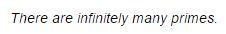# Infinitude of Primes - A. Thue's Proof### Proof

As common, we asum that every integer $n\gt 1\;$ has prime factorization. Now suppose (aiming for a contradiction) that there are only $k\;$ prime numbes: $2,\;$ $3,\;$ $5,\ldots,p.\;$ Then it follows from prime factorization that every positive integer $n\gt 1\;$ can be written in the form

$n=2^{a_1}3^{a_2}\cdots p^{a_k}$

for integers $a_1,a_2,\ldots,a_k\ge 0.\;$ Obviously, if $n\lt 2^m\;$ then $a_1,a_2,\ldots,a_k\lt m.\;$ But the number of sequences of $k\;$ positive integers $a_i\lt m\;$ is just $m^k,\;$ because each term in the sequence takes $m\;$ different values: $0,\;$ $1,\ldots,m-1.$

Since $k\;$ is fixed, $m^k\lt 2^m,\;$ for $m\;$ sufficiently large. This means that, for $m\;$ sufficiently large, there are not enough sequences $a_1,a_2,\ldots,a_k\;$ to represent all the numbers $n\lt 2^m\;$ in the form $2^{a_1}3^{a_2}\cdots p^{a_k},\;$ so we have a contradiction.

### Note

Thue's proof is dated 1897. There is a much more recent proof, with essentially the same underlying idea.

### References

1. J. Stillwell, Elements of Mathematics: From Euclid to Gödel, Princeton University Press, 2016, p 244